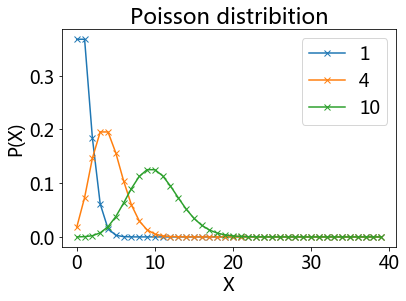# Distribution Functions¶## Function Definition¶

The following function will be used to show the different distributions functions

• Normal distribution

• Exponential distribution

• T-distribution

• F-distribution

• Logistic distribution

• Lognormal distribution

• Uniform distribution

• Binomial distribution

• Poisson distribution

# Note: here I use the iPython approach, which is best suited for interactive work
%pylab inline
from scipy import stats
matplotlib.rcParams.update({'font.size': 18})

Populating the interactive namespace from numpy and matplotlib

x = linspace(-10,10,201)
def showDistribution(d1, d2, tTxt, xTxt, yTxt, legendTxt, xmin=-10, xmax=10):
'''Utility function to show the distributions, and add labels and title.'''
plot(x, d1.pdf(x))
if d2 != '':
plot(x, d2.pdf(x), 'r')
legend(legendTxt)
xlim(xmin, xmax)
title(tTxt)
xlabel(xTxt)
ylabel(yTxt)
show()


## Normal distribution¶

Carl Friedrich Gauss discovered the normal distribution in 1809 as a way to rationalize the method of least squares.

The standard normal distribution is a special case when $\mu=0$ and $\sigma =1$, and it is described by this probability density function:

$$\varphi(x) = \frac 1{\sqrt{2\pi}}e^{- \frac 12 x^2}$$

• The factor $1/\sqrt{2\pi}$ in this expression ensures that the total area under the curve $\varphi(x)$ is equal to one.

• The factor $1/2$ in the exponent ensures that the distribution has unit variance (i.e., the variance is equal to one), and therefore also unit standard deviation.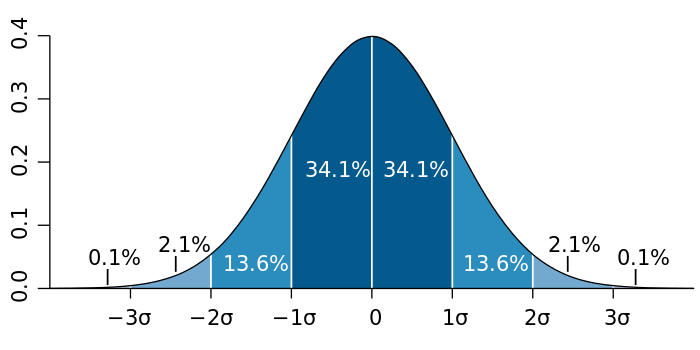showDistribution(stats.norm, stats.norm(loc=2, scale=4),
'Normal Distribution', 'Z', 'P(Z)','')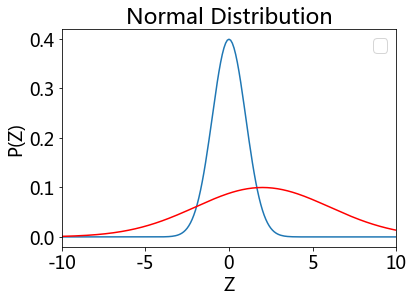### Exponential distribution¶

The probability density function (pdf) of an exponential distribution is

$$f(x;\lambda) = \begin{cases} \frac{1}{\beta} e^{-\frac{1}{\beta} x} & x \ge 0, \ 0 & x < 0. \end{cases}$$

$\beta$ is the scale parameter. Here $\frac{1}{\beta}$ is often called the rate parameter.

xs = range(10)
ys = [exp(-i) for i in xs]
ys2 = [1/2*exp(-1/2*i) for i in xs]
ys4 = [1/4*exp(-1/4*i) for i in xs]
plt.plot(xs, ys, label = 'beta = 1')
plt.plot(xs, ys2, label = 'beta = 2')
plt.plot(xs, ys4, label = 'beta = 4')
plt.legend();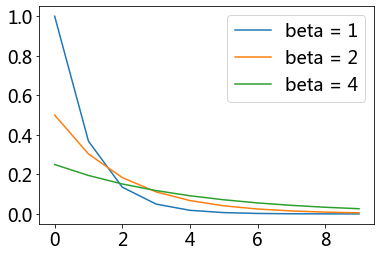# Exponential distribution
showDistribution(stats.expon(loc=0, scale = 1), stats.expon(loc=0, scale=4),
'Exponential Distribution', 'X', 'P(X)',['scale = 1', 'scale = 4'])## Students’ T-distribution¶

William Sealy Gosset

Let $X_1, \ldots, X_n$ be independent and identically distributed as $N(\mu, \sigma^2)$, i.e. this is a sample of size $n$ from a normally distributed population with expected mean value $\mu$ and variance $\sigma^2$.

• Let $\bar X = \frac 1 n \sum_{i=1}^n X_i$ be the sample mean

• let $S^2 = \frac 1 {n-1} \sum_{i=1}^n (X_i - \bar X)^2$ be the sample variance.

Then the random variable $\frac{ \bar X - \mu } { \sigma /\sqrt{n}}$ has a standard normal distribution (i.e. normal with expected mean 0 and variance 1),

The random variable $\frac{ \bar X - \mu} {S /\sqrt{n}},$ has a Student’s t-distribution with $n - 1$ degrees of freedom.

• where $S$ has been substituted for $\sigma$.

Student’s t-distribution has the probability density function given by

$f(t) = \frac{\Gamma(\frac{\nu+1}{2})} {\sqrt{\nu\pi},\Gamma(\frac{\nu}{2})} \left(1+\frac{t^2}{\nu} \right)^{!-\frac{\nu+1}{2}},!$

where $\nu$ is the number of degrees of freedom and $\Gamma$ is the gamma function.

This may also be written as

$f(t) = \frac{1}{\sqrt{\nu},\mathrm{B} (\frac{1}{2}, \frac{\nu}{2})} \left(1+\frac{t^2}{\nu} \right)^{!-\frac{\nu+1}{2}}!,$

where B is the Beta function.

As the number of degrees of freedom grows, the t-distribution approaches the normal distribution with mean 0 and variance 1.

For this reason ${\nu}$ is also known as the normality parameter.

# ... with 4, and with 10 degrees of freedom (DOF)
plot(x, stats.norm.pdf(x), 'g')
showDistribution(stats.t(4), stats.t(10),
'T-Distribution', 'X', 'P(X)',['normal', 't=4', 't=10'])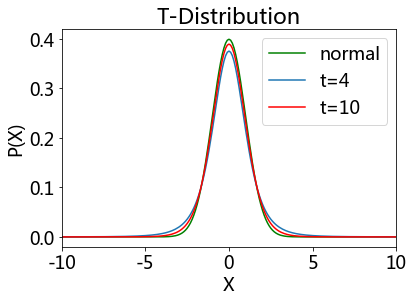## F-distribution¶

If a random variable X has an F-distribution with parameters d 1 and d 2, we write X ~ F(d1, d2). Then the probability density function (pdf) for X is given by

\begin\left\{align\right\} f\left(x; d_1,d_2\right) &= \frac\left\{\sqrt\left\{\frac\left\{\left(d_1x\right)^\left\{d_1\right\}\,\,d_2^\left\{d_2\right\}\right\} \left\{\left(d_1x+d_2\right)^\left\{d_1+d_2\right\}\right\}\right\}\right\} \left\{x\,\mathrm\left\{B\right\}\!\left\left(\frac\left\{d_1\right\}\left\{2\right\},\frac\left\{d_2\right\}\left\{2\right\}\right\right)\right\} \\ &=\frac\left\{1\right\}\left\{\mathrm\left\{B\right\}\!\left\left(\frac\left\{d_1\right\}\left\{2\right\},\frac\left\{d_2\right\}\left\{2\right\}\right\right)\right\} \left\left(\frac\left\{d_1\right\}\left\{d_2\right\}\right\right)^\left\{\frac\left\{d_1\right\}\left\{2\right\}\right\} x^\left\{\frac\left\{d_1\right\}\left\{2\right\} - 1\right\} \left\left(1+\frac\left\{d_1\right\}\left\{d_2\right\}\,x\right\right)^\left\{-\frac\left\{d_1+d_2\right\}\left\{2\right\}\right\} \end\left\{align\right\}

for real number x > 0. Here $\mathrm{B}$ is the beta function. In many applications, the parameters d 1 and d 2 are positive integers, but the distribution is well-defined for positive real values of these parameters.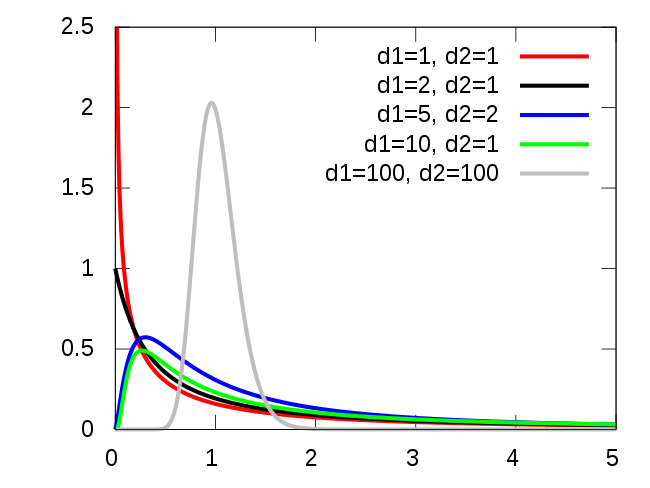# ... with (3,4) and (10,15) DOF
showDistribution(stats.f(3,4), stats.f(10,15),
'F-Distribution', 'F', 'P(F)',['(3,4) DOF', '(10,15) DOF'])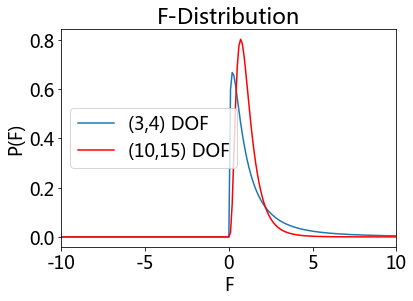## Uniform distribution¶

showDistribution(stats.uniform,'' ,
'Uniform Distribution', 'X', 'P(X)','')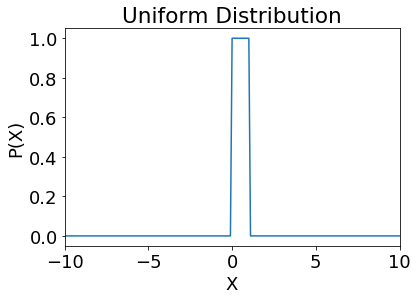## Logistic distribution¶

\begin\left\{align\right\} f\left(x; \mu,s\right) & = \frac\left\{e^\left\{-\left(x-\mu\right)/s\right\}\right\} \left\{s\left\left(1+e^\left\{-\left(x-\mu\right)/s\right\}\right\right)^2\right\} \\\left[4pt\right] & =\frac\left\{1\right\}\left\{s\left\left(e^\left\{\left(x-\mu\right)/\left(2s\right)\right\}+e^\left\{-\left(x-\mu\right)/\left(2s\right)\right\}\right\right)^2\right\} \\\left[4pt\right] & =\frac\left\{1\right\}\left\{4s\right\} \operatorname\left\{sech\right\}^2\left\left(\frac\left\{x-\mu\right\}\left\{2s\right\}\right\right). \end\left\{align\right\}

Because this function can be expressed in terms of the square of the hyperbolic function “sech”, it is sometimes referred to as the sech-square(d) distribution.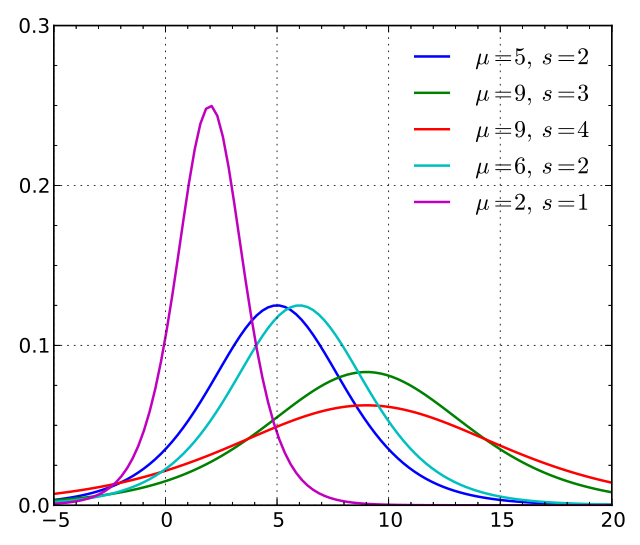showDistribution(stats.norm, stats.logistic,
'Logistic Distribution', 'X', 'P(X)',['Normal', 'Logistic'])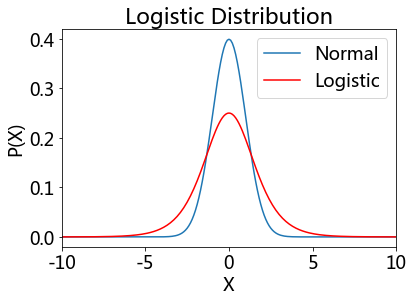## Lognormal distribution¶

A positive random variable X is log-normally distributed if the logarithm of X is normally distributed, $\ln(X) \sim \mathcal N(\mu,\sigma^2).$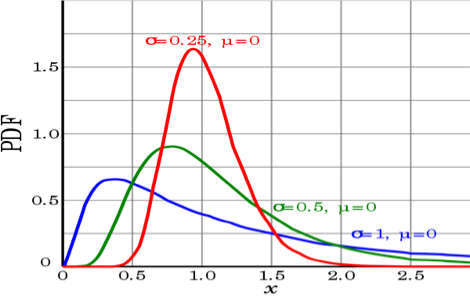x = logspace(-9,1,1001)+1e-9
showDistribution(stats.lognorm(2), '',
'Lognormal Distribution', 'X', 'lognorm(X)','', xmin=-0.1)# The log-lin plot has to be done by hand:
plot(log(x), stats.lognorm.pdf(x,2))
xlim(-10, 4)
title('Lognormal Distribution')
xlabel('log(X)')
ylabel('lognorm(X)')

<matplotlib.text.Text at 0x1f2d47ca518>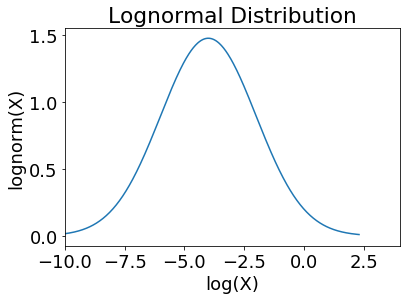## Binomial Distribution¶

The probability of getting exactly $k$ successes in $n$ independent Bernoulli trials is given by the probability density function:

$$f(k,n,p) = \Pr(k;n,p) = \Pr(X = k) = \binom{n}{k}p^k(1-p)^{n-k}$$

for k  = 0, 1, 2, …,  n, where $\binom{n}{k} =\frac{n!}{k!(n-k)!}$ is the binomial coefficient

• $k$ successes occur with probability pk

• $n-k$ failures occur with probability (1 − p)n − k.

bd1 = stats.binom(20, 0.5)
bd2 = stats.binom(20, 0.7)
bd3 = stats.binom(40, 0.5)
k = arange(40)
plot(k, bd1.pmf(k), 'o-b')
plot(k, bd2.pmf(k), 'd-r')
plot(k, bd3.pmf(k), 's-g')
title('Binomial distribition')
legend(['p=0.5 and n=20', 'p=0.7 and n=20', 'p=0.5 and n=40'])
xlabel('X')
ylabel('P(X)');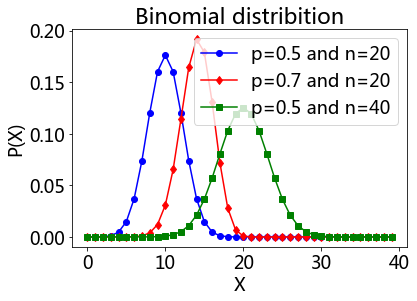## Poisson Distribution¶

The Poisson distribution is popular for modeling the number of times an event occurs in an interval of time or space.

A discrete random variable X is said to have a P\$oisson distribution with parameter λ > 0, if, for k = 0, 1, 2, …, the probability mass function of X is given by:

$$!f(k; \lambda)= \Pr(X = k)= \frac{\lambda^k e^{-\lambda}}{k!},$$

The positive real number λ is equal to the expected value of X and also to its variance

$$\lambda=\operatorname{E}(X)=\operatorname{Var}(X).$$

pd = stats.poisson(1)
pd4 = stats.poisson(4)
pd10 = stats.poisson(10)
plot(k, pd.pmf(k),'x-', label = '1')
plot(k, pd4.pmf(k),'x-', label = '4')
plot(k, pd10.pmf(k),'x-', label = '10')
title('Poisson distribition')
xlabel('X')
ylabel('P(X)')
legend();